Which of the following gates will have an output of 1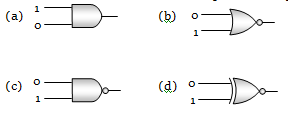Concept Questions :-

Logic gates
High Yielding Test Series + Question Bank - NEET 2020

Difficulty Level:

Which represents NAND gate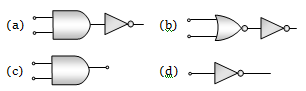Concept Questions :-

Logic gates
High Yielding Test Series + Question Bank - NEET 2020

Difficulty Level:

What will be the input of A and B for the Boolean expression $\overline{\left(\mathrm{A}+\mathrm{B}\right)}·\left(\overline{\mathrm{A}·\mathrm{B}}\right)=1$
(a) 0, 0                            (b) 0, 1
(c) 1, 0                            (d) 1, 1

Concept Questions :-

Logic gates
High Yielding Test Series + Question Bank - NEET 2020

Difficulty Level:

If A and B are two inputs in AND gate, then AND gate has an output of 1 when the values of A and B are
(a) A = 0, B = 0                         (b) A = 1, B = 1
(c) A = 1, B = 0                         (d) A = 0, B = 1

Concept Questions :-

Logic gates
High Yielding Test Series + Question Bank - NEET 2020

Difficulty Level:

The Boolean equation of NOR gate is
(a) C = A + B               (b) $\mathrm{C}=\overline{\mathrm{A}+\mathrm{B}}$
(c) $C=A·B$                 (d) $\mathrm{C}=\overline{\mathrm{A}.\mathrm{B}}$

Concept Questions :-

Logic gates

Difficulty Level:

This symbol represents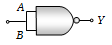(a) NAND gate
(b) OR gate
(c) AND gate
(d) NOR gate

Concept Questions :-

Logic gates

Difficulty Level:

Symbol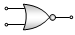(a) NAND gate                  (b) NOR gate
(c) NOT gate                     (d) XNOR gate

Concept Questions :-

Logic gates
High Yielding Test Series + Question Bank - NEET 2020

Difficulty Level:

To get an output 1 from the circuit shown in the figure, the input must be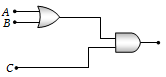(a) A = 0, B = 1, C = 0
(b) A = 1, B = 0, C = 0
(c) A = 1, B = 0, C = 1
(d) A = 1, B = 1, C = 0

Concept Questions :-

Logic gates
High Yielding Test Series + Question Bank - NEET 2020

Difficulty Level:

The combination of the gates shown in the figure below produces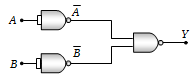(a) NOR gate
(b) OR gate
(c) AND gate
(d) XOR gate

Concept Questions :-

Logic gates
High Yielding Test Series + Question Bank - NEET 2020

Difficulty Level:

Which logic gate is represented by the following combination of logic gates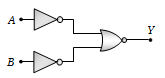(a) OR             (b) NAND
(c) AND           (d) NOR

Concept Questions :-

Logic gates